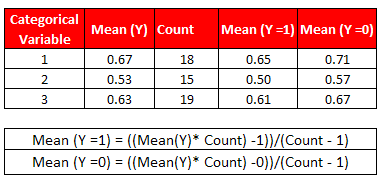# R Function : Convert Categorical Variables to Continuous Variables

In classification models, we generally encounter a situation when we have too many categories or levels in independent variables. The simple solution is to convert the categorical variable to continuous and use the continuous variables in the model. The easiest way to convert categorical variables to continuous is by replacing raw categories with the average response value of the category.

Adjusted Mean Value for Categorical Predictor

To have a different value against Y=1 and Y=0 for a categorical predictor, we can adjust the average response value of the category,Convert Categorical Variables to Continuous Variables

R Function: Converting Categorical Variables to Continuous
# Creating dummy data
set.seed(123)
mydata = data.frame(y= ifelse(sign(rnorm(100))==-1,0,1),
x1= sample(LETTERS[1:5],100,replace = TRUE),
x2= factor(sample(1:7, 100, replace = TRUE)))

# Convert categorical variables to continuous variables
TransformCateg <-  function(y,x,inputdata,cutoff){
for (i in seq(1,length(x),1)) {
if (class(inputdata[,x[i]]) %in% c("factor", "character")){
len <- NULL
t1 <- aggregate(inputdata[,y], list(inputdata[,x[i]]), mean)
names(t1) <- "avg"
t2 <- aggregate(inputdata[,y], list(inputdata[,x[i]]), length)
names(t2) <- "len"
temp <- merge(t1, t2, by = "Group.1")
t1 <- subset(temp, len >= cutoff)
t2 <- subset(temp, len < cutoff)
if(nrow(t2) > 0)
{
t2\$avg <- sum(t2\$avg*t2\$len)/sum(t2\$len)
t2\$len <- sum(t2\$len)
}
temp <- rbind(t1, t2)
inputdata <- merge(inputdata, temp, by.x = x[i], by.y = "Group.1", all.x = T)
inputdata[,paste(x[i],"mean", sep="_")] <- ((inputdata\$avg * inputdata\$len) - (inputdata[,y]))/(inputdata\$len - 1)
inputdata <- inputdata[, !(colnames(inputdata) %in% c("avg","len"))]
}
else{
warning(paste(x[i], " is not a factor or character variable", sep = ""))
}
}
return(inputdata)
}
# Run Function
train2 = TransformCateg(y= "y",x= c("x1","x2"), inputdata = mydata, cutoff = 15)
Parameters of TransformCateg Function

1. y : Response or target or dependent variable - categorical or continuous
2. x : a list of independent variables or predictors - Factor or Character Variables
3. inputdata : name of input data frame
4. cutoff : minimum observations in a category. All the categories having observations less than the cutoff will be a different category.

R Script : WOE Transformation of Categorical VariablesShare
Related PostsDeepanshu founded ListenData with a simple objective - Make analytics easy to understand and follow. He has over 10 years of experience in data science. During his tenure, he has worked with global clients in various domains like Banking, Insurance, Private Equity, Telecom and Human Resource.

4 Responses to "R Function : Convert Categorical Variables to Continuous Variables"
1.2.3.not continuous , discrete variables.

4.Thanks, though not clear

Next → ← Prev